# 2HDM + Scalar

Two Higgs-doublet models (2HDM) consisting of two Higgs fields are a particularly simple extension of the SM, which can be found in many well-motivated theories.  Adding a scalar singlet to a 2HDM provides a wealth of possible exotic Higgs decays.  A comprehensive review of 2HDM can be found in , while for a shorter summary we refer the reader to Section 1.3.2 of arXiv:1312.4992.

The phenomenology of the 2HDM models is generally dictated by two parameters : tanβ = v2/v1 (v1, v2 are vacuum expectation values of the Higgs doublets) and the rotation angle α that defines the scalar mass eigenstates in terms of the real components of the doublets. To avoid large Flavor-Changing Neutral Currents (FCNCs), Z2 symmetries can be imposed to ensure that fermions with the same quantum numbers all couple to only one Higgs field. This results in four "standard" types of fermion couplings commonly discussed in the literature (see Section 1.3.2 and Table II of arXiv:1312.4992).

One real mass eigenstate, h, is identified as the  ∼ 125  GeV SM-like Higgs, while other states of the 2HDM are taken to be heavy.  This is easily achieved in the decoupling limit,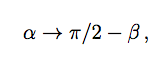The decoupling limit also ensures that highly non-standard Higgs decays in 2HDM, which are significantly constrained by existing data  [2,3], are avoided.   The scalar singlet that was added to the 2HDM only couples to the two Higgs fields from the 2HDM (H1,2) in the potential and has no direct Yukawa couplings, acquiring all of its couplings to SM fermions through its mixing with H1,2. This mixing needs to be small to avoid spoiling the SM-like nature of h.

Under these simple assumptions, exotic Higgs decays of the form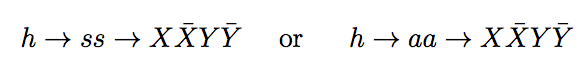as well as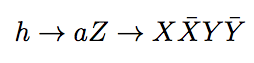are possible, where s(a) is a (pseudo)scalar mass eigenstate mostly composed of the real (imaginary) parts of the singlet field, and X, Y are SM fermions or gauge bosons. We refer to this setup as the 2HDM+S. For "Type II" 2HDM+S, a light a can correspond to the R-symmetry limit of the NMSSM. However, a more general 2HDM+S framework allows for exotic Higgs decay phenomenologies that are much more diverse than those usually considered in an NMSSM-type setup.

To incorporate the already analyzed constraints on 2HDM's into the 2HDM+S (e.g. ), one can imagine adding a decoupled singlet sector to a 2HDM with α, β chosen so as to not yet be excluded. The real and imaginary components of S can be given separate masses, and small mixings to the 2HDM sector can then be introduced as a perturbation. Approximately the same constraints on α, β apply to this 2HDM+S, as long as Br(hss/aa/Za) <~10%. This allows for a wide range of possible exotic Higgs decays. There are some important differences depending on 1) whether the lightest singlet state with a mass below mh/2 is scalar or pseudo scalar 2) whether the lightest singlet state mass is above or below chiral symmetry breaking scale, which are discussed in detail in Section 1.3.2 and Appendix A of arXiv:1312.4992, respectively.  The relevant branching ratio calculations are also given in Appendix A of the same document. To illustrate the rich decay phenomenology of 2HDM+S models, we plot below the branching ratios of a pseudoscalar to SM particles in Fig. 1 and Fig. 2 for Type II and Type III (lepton-specific) 2HDM+S models, respectively.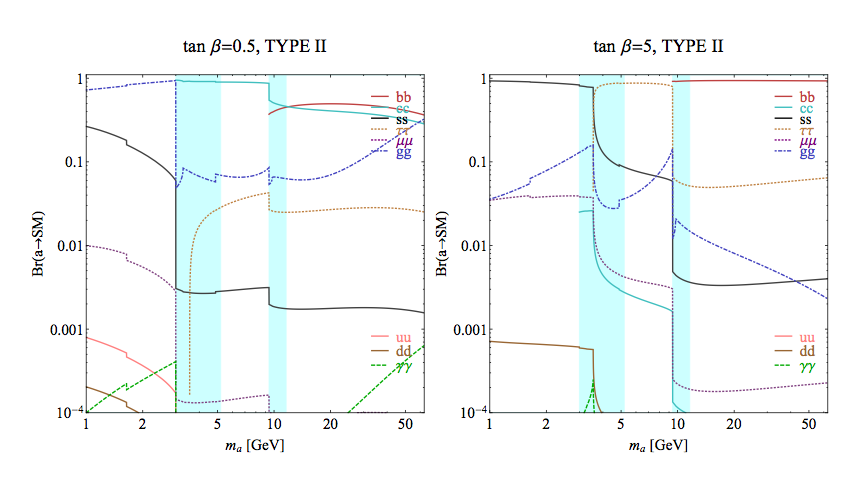Figure 1: Branching ratios of a singlet-like pseudoscalar in the 2HDM+S for Type II Yukawa couplings. Decays to quarkonia likely invalidate our simple calculations in the shaded regions.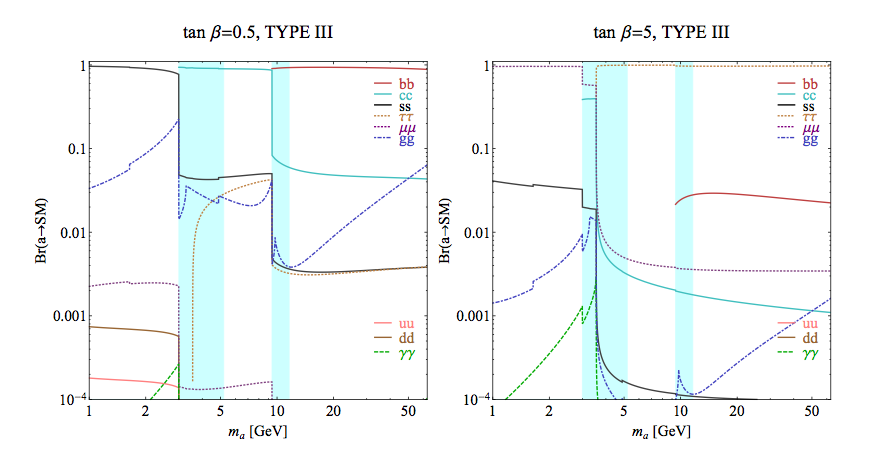Figure 2: Branching ratios of a singlet-like pseudoscalar in the 2HDM+S for Type III Yukawa couplings. Decays to quarkonia likely invalidate our simple calculations in the shaded regions.